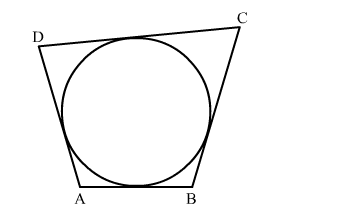# Quadrilateral ABCD is circumscribed to a circle.`
Question:

Quadrilateral ABCD is circumscribed to a circle. If AB = 6 cm, BC = 7 cm and CD = 4 cm, then the length of AD is

(a) 3 cm
(b) 4 cm
(c) 6 cm
(d) 7 cm

Solution:We know that when a quadrilateral circumscribes a circle then sum of opposites sides is equal to the sum of other opposite sides.
∴ AB + DC = AD + BC
⇒6 + 4 = AD + 7